# cos'x dx sin 3x dx 2. an 45 sin cos'xdx 4 sin'xcos'x dr 44 sin'x cos'r...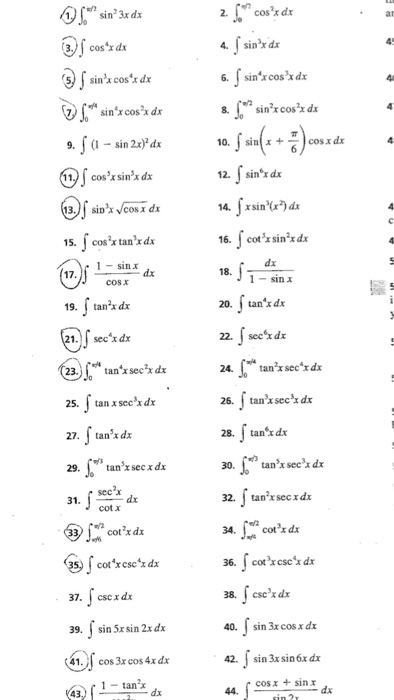cos'x dx sin 3x dx 2. an 45 sin cos'xdx 4 sin'xcos'x dr 44 sin'x cos'r dr 6. sin'xcosx dx 8. Jo sin'x cosx dx fa-sin 2x)' dx sin x + cos x dx 10. 9 f sin'z dx cos'x sin'x d 12. 11 sin'x Vcosx dx 14. 13. cot'r sin'x dx 16. cos'x tan'xdx 15 dx sin x dx 18. 17 1-sin x cos x tan'x dx 20. tanx dx 19 sec'x d sec'x dx 22. 21 tan'x secxdx tan'x secx dx 24. (23. tan'x sec' dx 26. tan x sec'x dx 25. tan'x dx tan'x d 28. 27. tan'x sec x dx 29. Jo tan'x secx dx 30. secx dx cot x tan'x secx ds 32. 31 cot'x dx cot'x dx 34. 33 cor'xese's dx 35cot'xcsc' dx 36. ese'x dx 38. 37 cse x dx sin 3x cosx dx 40. sin 5r sin 2x dx 39. sin 3x sin 6x dx 42. 41 cos 3x cos 4x dx cos x sinx dx sin 2 1 tanx dx 43) 44.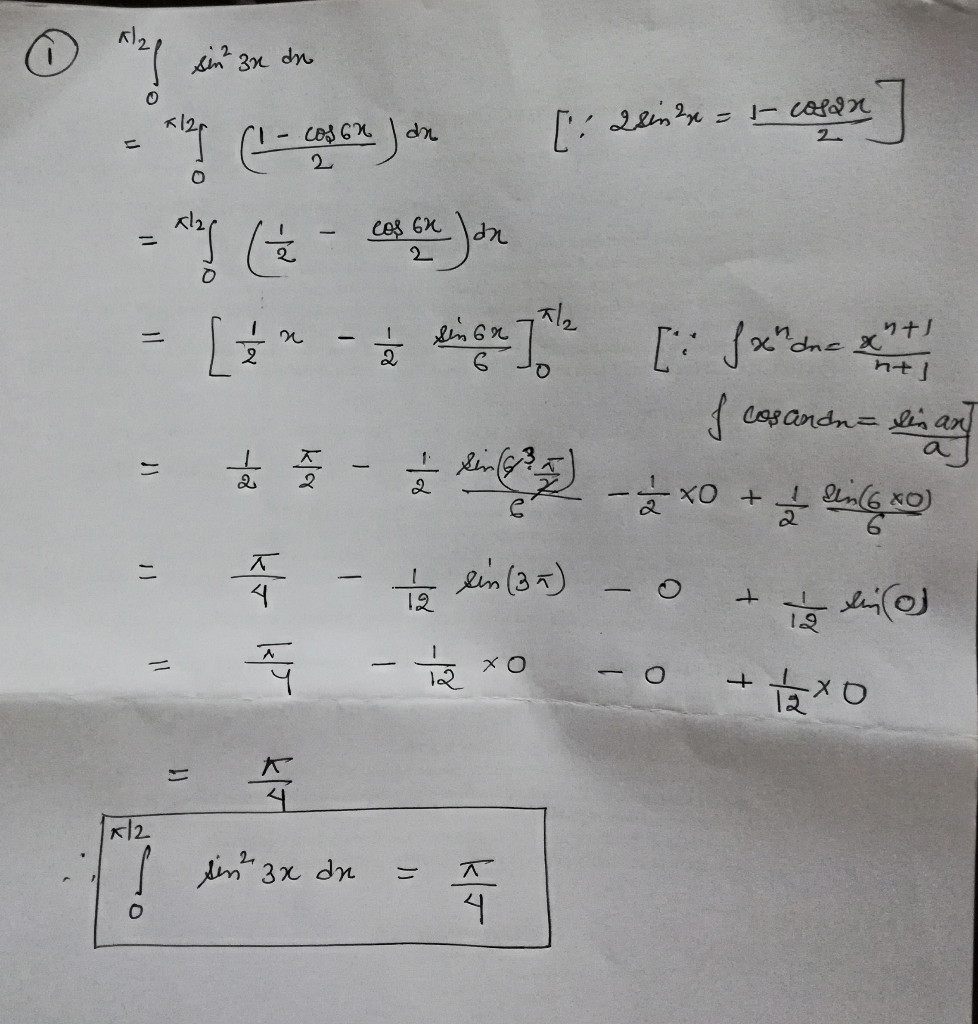##### Add Answer of: cos'x dx sin 3x dx 2. an 45 sin cos'xdx 4 sin'xcos'x dr 44 sin'x cos'r...
Similar Homework Help Questions
• ### Create an algebraic expression for sin(arccosx-arcsin3x) 2

1. Create an algebraic expression for sin(arccosx-arcsin3x)2. The cosx=4/5, x lies in quadrant 4. Find sin x/23.Determine all solutions in (0,2pie) for sin4xsin^2x=3,cot^2v(3-1)cotx=v(3), cos^2x=cosx, andtan^2x-6tanx+4=04. Solve; sin(∏/2-x)=1/sec5. Solve; cot+4x+2cot^2x+1=csc4x6. Solve; tan(x+∏/x=(√(3)+3tanx)/(3-√(3)tanx

• ### i have a few questions cosx + cosx =2secx ----- ----- 1+sinx 1-sinx cos(x-B)-cos(x-B) = 2sinxsinB csc2x= 1 secx cscx --- 2 cotx= cos5x+cos3x ----------- sin 5x-sin 3x

i have a few questions cosx + cosx =2secx----- -----1+sinx 1-sinxcos(x-B)-cos(x-B) = 2sinxsinBcsc2x= 1 secx cscx --- 2cotx= cos5x+cos3x ----------- sin 5x-sin 3x

• ### 8. (a) (5pt) Prove the identity: cscx -cos x=sec x . sin'x 4 and tan θ<0 (b) (5pt) Find cos θ , if sin θ 8. (a) (5pt) Prove the identity: cscx -cos x=sec x . sin'x 4 and tan θ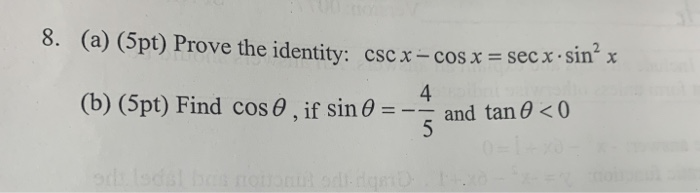8. (a) (5pt) Prove the identity: cscx -cos x=sec x . sin'x 4 and tan θ<0 (b) (5pt) Find cos θ , if sin θ 8. (a) (5pt) Prove the identity: cscx -cos x=sec x . sin'x 4 and tan θ

• ### verify that each of the following is an identity: 1) cos x/ 1-sin^2x= sec x 2) sec x/sin x - sin x/cos x = cot x 3) 1+tan^2ø/ cos ^2ø = sec^4 ø I really need ur help :) thank u so much

verify that each of the following is an identity: 1) cos x/ 1-sin^2x= sec x 2) sec x/sin x - sin x/cos x = cot x 3) 1+tan^2ø/ cos ^2ø = sec^4 ø I really need ur help :) thank u so much

• ### (f) If a and b are unit vectors and θ is the angle between them. prove that sin -b Prove that v2·“2 + 2,6 , where the symbol's h) have their usual meanings 12. Find the following dx dx tan'xd...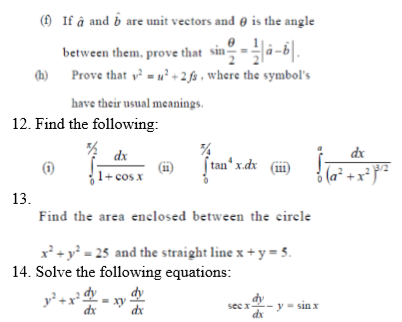(f) If a and b are unit vectors and θ is the angle between them. prove that sin -b Prove that v2·“2 + 2,6 , where the symbol's h) have their usual meanings 12. Find the following dx dx tan'xdx (i) 1+cos x 13 Find the area enclosed between the circle 2y25 and the straight line x +y- s 14. Solve the following equations dy dy dy dx (f) If a and b are unit vectors and θ is the...

• ### Find dy/dx of the next relations      Sol: y ylx 1 1-Cx C 2) 1+cx y' (1+cx1-cx a+bx ab 3) y= In Va-bx y's a2-b&#...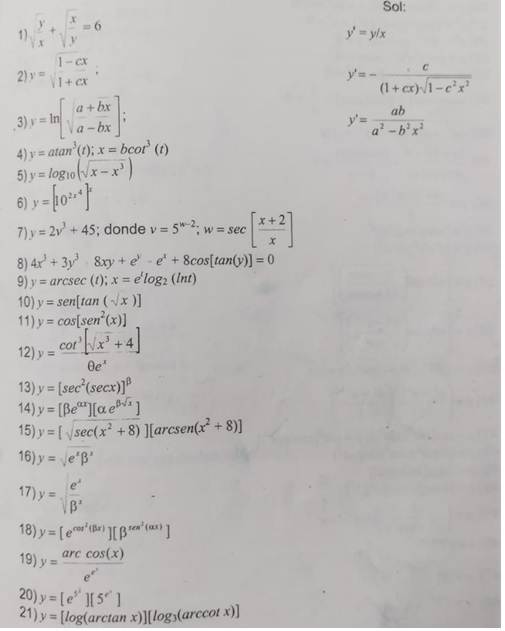Find dy/dx of the next relations      Sol: y ylx 1 1-Cx C 2) 1+cx y' (1+cx1-cx a+bx ab 3) y= In Va-bx y's a2-b'x 4) y= atan (t); x = bcor (t) 6) x+2 7) y = 2v+ 45; donde v 52., W sec X 8)4x+3 8xy+e -e+ 8cos[tan(y)] = 0 arcsec (); x = elog2 (Int) 9) y 10) y sen[tan(x )] 11) y = cos[sen' (x)] cot + 4 12) y 13) y = [sec'(secx))P 14) y [Beae...

• ### find the indefinite integral and check the result by differentiation Analytic Geometry & Calculus II, Final Examination Part I, Spring costx)swLx) b) J sin 2x cos 2x dx 2 (-cos (4)(Hx) chec...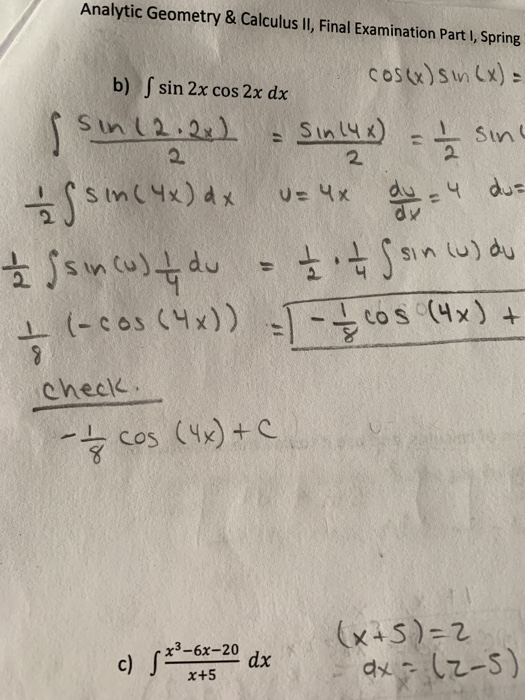find the indefinite integral and check the result by differentiation Analytic Geometry & Calculus II, Final Examination Part I, Spring costx)swLx) b) J sin 2x cos 2x dx 2 (-cos (4)(Hx) check. (x-5)=2 xs_6x-20 dx Analytic Geometry & Calculus II, Final Examination Part I, Spring costx)swLx) b) J sin 2x cos 2x dx 2 (-cos (4)(Hx) check. (x-5)=2 xs_6x-20 dx

• ### DO NOT use a calculator. Exact answers only, no decimals. 1. (10 pt each) Evaluate the following integrals: since) dz In(In(x b. dr c. cos(x)(sin(a)2 dz d.2tan (') dr 1. (10 pt each) Evalu...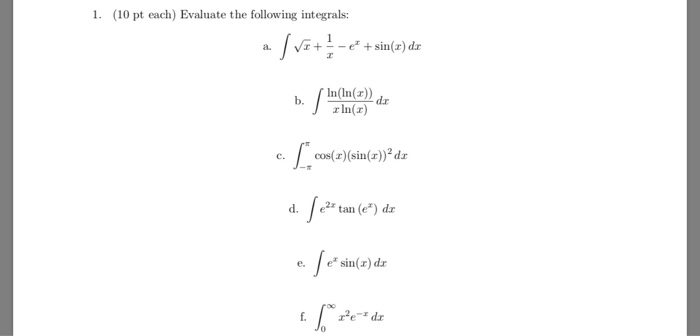DO NOT use a calculator. Exact answers only, no decimals. 1. (10 pt each) Evaluate the following integrals: since) dz In(In(x b. dr c. cos(x)(sin(a)2 dz d.2tan (') dr 1. (10 pt each) Evaluate the following integrals: since) dz In(In(x b. dr c. cos(x)(sin(a)2 dz d.2tan (') dr

• ### A) Find the solution of the given 2nd order Homogenous ODE using undetermined coefficient 1) y"-10y, + 25y-30x + 3 4 3) y"- 16y - 2e4x 4) y" + 2y'ysin x + 3 cos 2x B) Find the sol...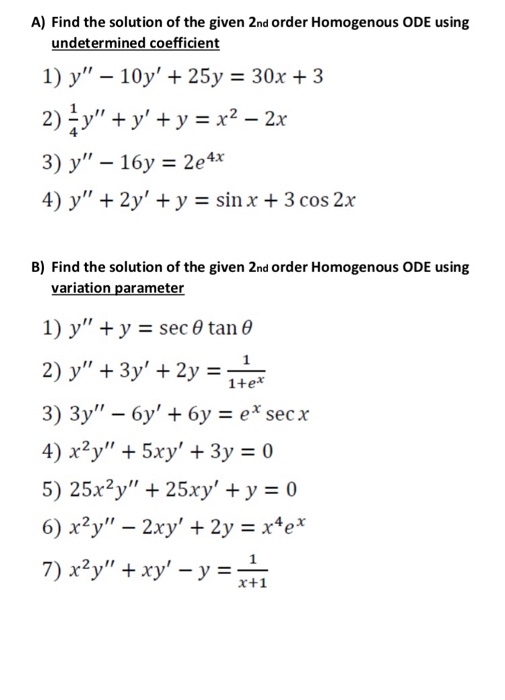A) Find the solution of the given 2nd order Homogenous ODE using undetermined coefficient 1) y"-10y, + 25y-30x + 3 4 3) y"- 16y - 2e4x 4) y" + 2y'ysin x + 3 cos 2x B) Find the solution of the given 2nd order Homogenous ODE using variation parameter 1) y" + y sec θ tan θ 1+e 3) 3y''-6y' + 6y = ex secx x+1 A) Find the solution of the given 2nd order Homogenous ODE using undetermined coefficient...

• ### i have some problems doing trig the first one is: Show that cos(x/2) sin(3x/2) = ½(sinx + sin2x) i know that you are supposed to substitute all those trig function things in it but i kind of forgot how to the only that i can see substituting in is the

i have some problems doing trig the first one is: Show that cos(x/2) sin(3x/2) = ½(sinx + sin2x) i know that you are supposed to substitute all those trig function things in it but i kind of forgot how to the only that i can see substituting in is the double angle one for sin2x could anyone walk me through the process maybe?What a very nasty trig identity. I am sure this is not the most efficient way, but the...

Need Online Homework Help?